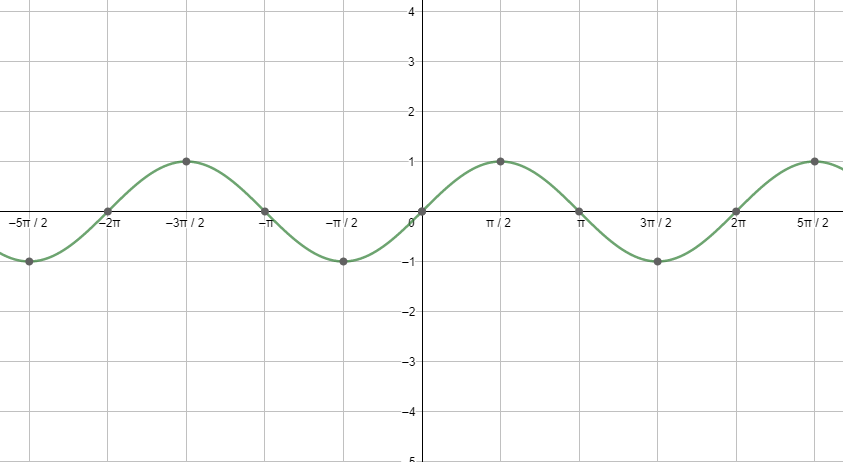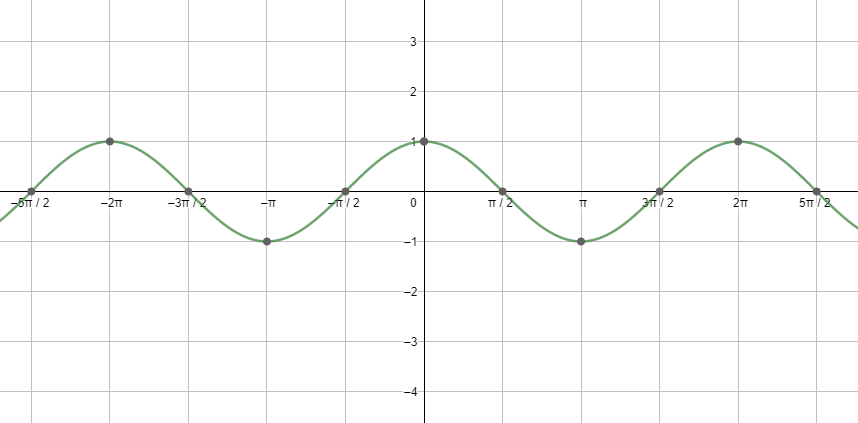QuestionAnswers

# If $x=\cot A+\cos A$ and $y=\cot A-\cos A$ . Then prove that ${{\left( \dfrac{x-y}{x+y} \right)}^{2}}+{{\left( \dfrac{x-y}{2} \right)}^{2}}=1$ .Hint: Try to simplify the left-hand side of the equation that we need to prove by substituting x and y with their respective values as given in the question. Finally, use the formula ${{\sin }^{2}}x+{{\cos }^{2}}x=1$ to reach the desired result.

Before moving to the solution, let us discuss the periodicity of sine and cosine function, which we would be using in the solution. All the trigonometric ratios, including sine and cosine, are periodic functions. We can better understand this using the graph of sine and cosine.Next, let us see the graph of cosx.Looking at both the graphs, we can say that the graphs are repeating after a fixed period i.e. $2{{\pi }^{c}}$ . So, we can say that the fundamental period of the cosine function and the sine function is $2{{\pi }^{c}}=360{}^\circ$

We will now solve the left-hand side of the equation given in the question.

${{\left( \dfrac{x-y}{x+y} \right)}^{2}}+{{\left( \dfrac{x-y}{2} \right)}^{2}}$

Now we will substitute x and y as given in the question. On doing so, we get

${{\left( \dfrac{\cot A+\cos A-\left( \cot A-\cos A \right)}{\cot A+\cos A+\operatorname{cotA}-cosA} \right)}^{2}}+{{\left( \dfrac{\cot A+\cos A-\left( \cot A-\cos A \right)}{2} \right)}^{2}}$

$={{\left( \dfrac{\cot A+\cos A-\cot A+\cos A}{\cot A+\cos A+\operatorname{cotA}-cosA} \right)}^{2}}+{{\left( \dfrac{\cot A+\cos A-\cot A+\cos A}{2} \right)}^{2}}$

$={{\left( \dfrac{\cos A}{\cot A} \right)}^{2}}+{{\left( \dfrac{\cos A}{{}} \right)}^{2}}$

$={{\left( \dfrac{\cos A}{\cot A} \right)}^{2}}+{{\left( \cos A \right)}^{2}}$

Now we know that $\cot A=\dfrac{\cos A}{\sin A}$ . So, our equation becomes

${{\left( \dfrac{\sin A\cos A}{\cos A} \right)}^{2}}+{{\left( \cos A \right)}^{2}}$

$={{\left( \sin A \right)}^{2}}+{{\left( \cos A \right)}^{2}}$

We know that the sum of the squares of the sine and cosine function of an angle is equal to
1. So, our expression turns out to be:

${{\left( \sin A \right)}^{2}}+{{\left( \cos A \right)}^{2}}$

$=1$

As we have shown that the left-hand side of the equation given in the question is equal to the right-hand side of the equation in the question. Therefore, we can say that we have proved that ${{\left( \dfrac{x-y}{x+y} \right)}^{2}}+{{\left( \dfrac{x-y}{2} \right)}^{2}}=1$ .

Note: Be careful about the calculation and the signs while opening the brackets. The general mistake that a student can make is 1+x-(x-1)=1+x-x-1. Also, it would help if you remember the properties related to complementary angles and trigonometric ratios.

The Difference Between an Animal that is A Regulator and One that is A ConformerArea of a Sector of a Circle FormulaDrafting Minutes of a Meeting and ATRWhere There is a Will There is a Way EssayCBSE Class 8 Maths Chapter 9 - Algebraic Expressions and Identities FormulasMagnetic and Electric Force on a Point ChargeArea of a Rhombus FormulaPerimeter of a Trapezoid FormulaReflection on A Plane MirrorTransistor as a DeviceImportant Questions for CBSE Class 6 English A Pact with The Sun Chapter 10 - A Strange Wrestling MatchImportant Questions for CBSE Class 6 English A Pact with The Sun Chapter 8 - A Pact with the SunCBSE Class 7 Science Water: A Precious Resource WorksheetsImportant Questions for CBSE Class 6 English A Pact with The Sun Chapter 1 - A Tale of Two BirdsImportant Questions for CBSE Class 11 Physics Chapter 4 - Motion in a PlaneImportant Questions for CBSE Class 6 English A Pact with The SunImportant Questions for CBSE Class 6 English Honeysuckle Chapter 8 - A Game of ChanceImportant Questions for CBSE Class 11 Business Studies Chapter 7 - Formation of a CompanyImportant Questions for CBSE Class 8 English Honeydew Chapter 7 - A Visit to CambridgeImportant Questions for CBSE Class 7 Social Science - Social and Political Life Chapter 8 - A Shirt In The MarketCBSE Class 10 Hindi A Question Paper 2020Hindi A Class 10 CBSE Question Paper 2009Hindi A Class 10 CBSE Question Paper 2015Hindi A Class 10 CBSE Question Paper 2016Hindi A Class 10 CBSE Question Paper 2012Hindi A Class 10 CBSE Question Paper 2010Hindi A Class 10 CBSE Question Paper 2008Hindi A Class 10 CBSE Question Paper 2014Hindi A Class 10 CBSE Question Paper 2007Hindi A Class 10 CBSE Question Paper 2013RS Aggarwal Class 8 Mathematics Solutions for Chapter-18 Area of a Trapezium and a PolygonRS Aggarwal Solutions Class 8 Chapter-18 Area of a Trapezium and a Polygon (Ex 18B) Exercise 18.2NCERT Solutions for Class 12 English Kaliedoscope Poetry Chapter 4 Kubla Khan or a Vision in a Dream a FragmentRS Aggarwal Solutions Class 8 Chapter-18 Area of a Trapezium and a Polygon (Ex 18C) Exercise 18.3RS Aggarwal Solutions Class 8 Chapter-18 Area of a Trapezium and a Polygon (Ex 18A) Exercise 18.1NCERT Solutions for Class 6 English Honeysuckle Poem - A House, A HomeRD Sharma Class 12 Maths Solutions Chapter 7 - Adjoint and Inverse of a MatrixRS Aggarwal Class 10 Solutions - Trigonometric IdentitiesRD Sharma Solutions for Class 10 Maths Chapter 6 - Trigonometric IdentitiesNCERT Solutions for Class 9 English Moments Chapter 8 - A House is Not a Home